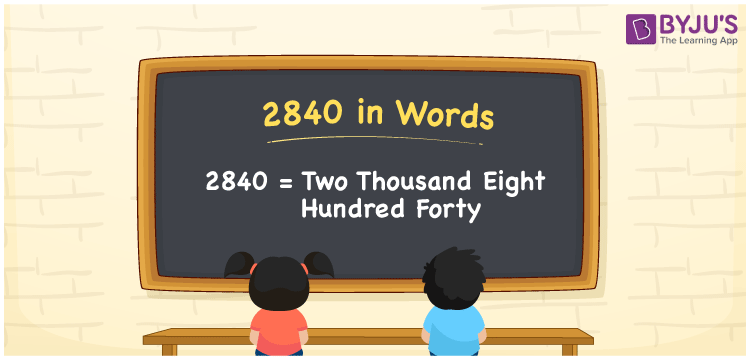# 2840 in Words

2840 in words can be written as Two Thousand Eight Hundred Forty. If you offer food to the orphanage for Rs. 2840, then you can say that “I offered food for Two Thousand Eight Hundred Forty Rupees”. You will obtain an in depth knowledge of numbers in words by referring to this article. Therefore, the number 2840 can be read as “Two Thousand Eight Hundred Forty” in words.

 2840 in words Two Thousand Eight Hundred Forty Two Thousand Eight Hundred Forty in Numbers 2840

## 2840 in English Words## How to Write 2840 in Words?

First determine the place value of each digit to write the number into words. The place value chart of 2840 is provided below.

 Thousands Hundreds Tens Ones 2 8 4 0

2840 in expanded form is:

2 x Thousand + 8 × Hundred + 4 × Ten + 0 × One

= 2 x 1000 + 8 × 100 + 4 × 10 + 0 × 1

= 2000 + 800 + 40

= 2840

= Two Thousand Eight Hundred Forty

Therefore, 2840 in words is written as Two Thousand Eight Hundred Forty.

2840 is a natural number that precedes 2841 and succeeds 2839.

2840 in words – Two Thousand Eight Hundred Forty

Is 2840 an odd number? – No

Is 2840 an even number? – Yes

Is 2840 a perfect square number? – No

Is 2840 a perfect cube number? – No

Is 2840 a prime number? – No

Is 2840 a composite number? – Yes

## Frequently Asked Questions on 2840 in Words

Q1

### Write 2840 in words.

2840 can be written in words as “Two Thousand Eight Hundred Forty”.
Q2

### How do you write Two Thousand Eight Hundred Forty in numbers?

Two Thousand Eight Hundred Forty can be written in numbers as 2840.
Q3

### Is 2840 an even number?

Yes, 2840 is an even number as it is completely divisible by 2.